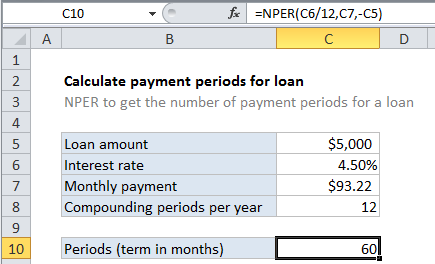## Excel Office

Excel How Tos, Tutorials, Tips & Tricks, Shortcuts

# Calculate payment periods for loan in Excel

To calculate the number of payment periods for a loan, given the loan amount, the interest rate, and a periodic payment amount, you can use the NPER function.

## Formula

`=NPER(rate,payment,-loan)`## Explanation

In the example shown, the formula in C10 is…

`=NPER(C6/12,C7,-C5)`

### How this formula works

Loans have four primary components: the amount, the interest rate, the number of periodic payments (the loan term) and a payment amount per period. One use of the NPER function is to calculate the number of periodic payments for loan.

Worked Example:   PMT, RATE, NPER, PV and FV Financial Functions in Excel

For this example, we want to calculate the number of payments for a \$5000 loan, with a 4.5% interest rate, and fixed payments of \$93.22. The NPER function is configured as follows:

rate – The interest rate per period. We divide the value in C6 by 12 since 4.5% represents annual interest:

`=C6/12`

pmt – The payment made each period. This is the known amount \$93.22, which comes from cell C7.

Worked Example:   NPER function: Description, Usage, Syntax, Examples and Explanation

pv – The present value, or total value of all payments now. In the case of a loan, this is input as a negative value by adding a negative sign in from of C5:

`-C5`

fv – the future value, or desired balance after last payment. We do not provide a value since the default is zero and makes sense for a loan.

Worked Example:   Calculate periods for annuity in Excel

type – When payments are due, where 0 = end of period and 1 = beginning of period. Default is 0 so we do not provide a value.

With these inputs, the NPER function returns 59.996, which is rounded to 60 in the example, representing 60 months = 5 years. The monthly payment is rounded to the nearest penny, which causes NPER to return a number slightly less than 60.